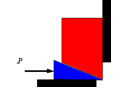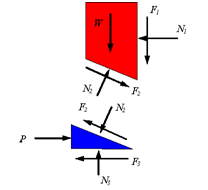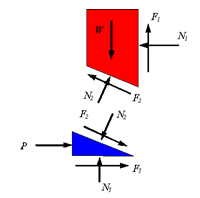Wedge Friction

# Wedge Friction Notes | Study Engineering Mechanics - Mechanical Engineering

## Document Description: Wedge Friction for Mechanical Engineering 2022 is part of Engineering Mechanics preparation. The notes and questions for Wedge Friction have been prepared according to the Mechanical Engineering exam syllabus. Information about Wedge Friction covers topics like and Wedge Friction Example, for Mechanical Engineering 2022 Exam. Find important definitions, questions, notes, meanings, examples, exercises and tests below for Wedge Friction.

Introduction of Wedge Friction in English is available as part of our Engineering Mechanics for Mechanical Engineering & Wedge Friction in Hindi for Engineering Mechanics course. Download more important topics related with notes, lectures and mock test series for Mechanical Engineering Exam by signing up for free. Mechanical Engineering: Wedge Friction Notes | Study Engineering Mechanics - Mechanical Engineering
 1 Crore+ students have signed up on EduRev. Have you?

Wedge: A wedge is in general a triangular object which is placed between two objects to either hold them in place or is used to move one relative to the other. For example, the following shows a wedge under a block that is supported by the wall.If the force P is large enough to push the wedge forward, then the block will rise and the following is an appropriate free-body diagram. Note that for the wedge to move one needs to have slip on all three surfaces. The direction of the friction force on each surface will oppose the slipping.Since before the wedge can move each surface must overcome the resistance to slipping, one can assume that

F1 =μN1

F2 =μN2

F3 = μN3

These equations and the equations of equilibrium are combined to solve the problem. If the force P is not large enough to hold the top block from coming down, then the wedge will be pushed to the left and the appropriate free-body diagram is the following. Note that the only change is the direction of the frictional forces. A similar analysis to the above yield the solution to the problem.The document Wedge Friction Notes | Study Engineering Mechanics - Mechanical Engineering is a part of the Mechanical Engineering Course Engineering Mechanics.
All you need of Mechanical Engineering at this link: Mechanical Engineering

## Engineering Mechanics

24 videos|71 docs|39 tests
 Use Code STAYHOME200 and get INR 200 additional OFF

## Engineering Mechanics

24 videos|71 docs|39 tests

Track your progress, build streaks, highlight & save important lessons and more!

,

,

,

,

,

,

,

,

,

,

,

,

,

,

,

,

,

,

,

,

,

;